# MP Board Class 6th Maths Solutions Chapter 7 Fractions Ex 7.5

## MP Board Class 6th Maths Solutions Chapter 7 Fractions Ex 7.5

Question 1.
Write these fractions appropriately as additions or subtractions: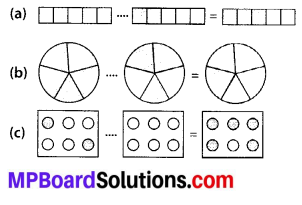Solution: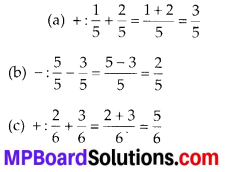Question 2.
Solve: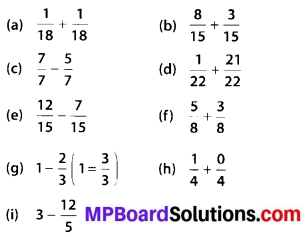Solution: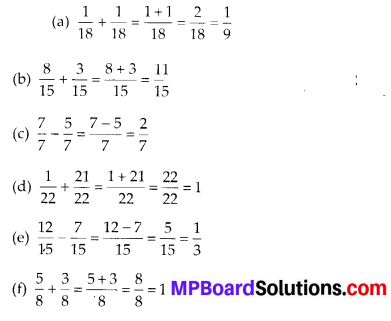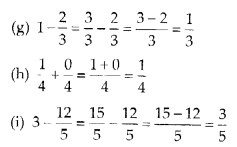Question 3.
Shubham painted $$\frac{2}{3}$$ of the wall space in his room. His sister Madhavi helped and painted $$\frac{1}{3}$$ of the wall space. How much did they paint together?
Solution:
Fraction of wall painted by Shubham = $$\frac{2}{3}$$
Fraction of wall painted by Madhavi = $$\frac{1}{3}$$
Total painting by both of them $$=\frac{2}{3}+\frac{1}{3}=\frac{2+1}{3}=\frac{3}{3}=1$$
Therefore, they painted complete wall.

Question 4.
Fill in the missing fractions.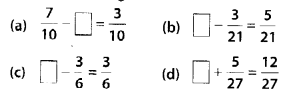Solution: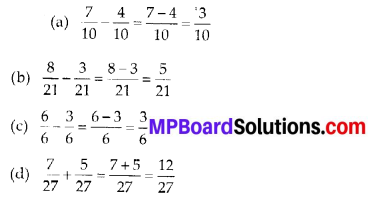Question 5.
Javed was given $$\frac{5}{7}$$ of a basket of oranges. What fraction of oranges was left in the basket?
Solution:
Consider the total number of oranges to be the whole portion or 1.
Fraction of oranges left = $$1-\frac{5}{7}$$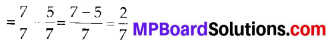Thus, $$\frac{2}{7}$$ oranges was left in the basket.